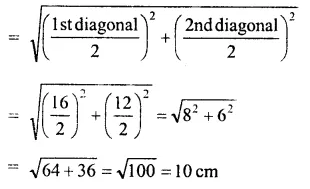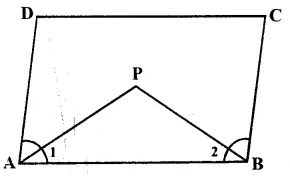## RS Aggarwal Class 8 Solutions Chapter 16 Parallelograms Ex 16B

These Solutions are part of RS Aggarwal Solutions Class 8. RS Aggarwal Solutions Class 8 Chapter 16 Parallelograms Ex 16B.

Other Exercises

Questions Tick the correct answer in each of the following.

Question 1.
Solution:
The diagonals of a rhombus are not necessarily equal but the diagonals in rectangle, square and isosceles trapezium are always equal.

Question 2.
Solution:
Each side of a rhombusQuestion 3.
Solution:
The sum of adjacent angles of a || gm = 180°
2x + 25° + 3x – 5° = 180°
=> 5x + 20° = 180°
=> 5x = 180° – 20° = 160°
=> x = $$\\ \frac { 160 }{ 5 }$$
= 32°

Question 4.
Solution:
The diagonals in rhombus, kite intersect each other at right angles.
But the diagonals of parallelogram do not necessarily intersect at right angles.

Question 5.
Solution:
Let l = 4x, b = 3x,
Then (diagonal)² = l² + b²
=> (25)² = 16x² + 9x²
=> 25x² = 625
=> x² = 25
=> x = 5
=> l = 4x = 4 x 5 = 20cm
b = 3x = 3 x 5 = 15cm
Perimeter = 2(l + b) = 2 (20 + 15)
= 2 x 35 = 70 cm

Question 6.
Solution:
AP and BP are the bisector of ∠A and ∠B
Sum of two adjacent angles of a ||gm = 180°
or ∠A + ∠B = 180°But ∠1 = $$\\ \frac { 1 }{ 2 }$$ ∠A and ∠2 =$$\\ \frac { 1 }{ 2 }$$ ∠B
∠1 + ∠2 = $$\\ \frac { 1 }{ 2 }$$ ∠A + $$\\ \frac { 1 }{ 2 }$$ ∠B
= $$\\ \frac { 1 }{ 2 }$$ (∠A + ∠B)
= 180° x $$\\ \frac { 1 }{ 2 }$$ = 90°
∠P = 180° – (∠1 + ∠2)
= 180° – 90° = 90°

Question 7.
Solution:
Let one adjacent angle = x
Then second angle (smallest) = $$\frac { 2 }{ 3 } x$$
x + $$\frac { 2 }{ 3 } x$$ = 180°
= $$\frac { 5 }{ 3 } x$$ = 180°
=> x = 180° x $$\\ \frac { 3 }{ 5 }$$ = 108°
=> Smallest angle = 108° x $$\\ \frac { 2 }{ 3 }$$ = 72°

Question 8.
Solution:
The diagonals of square, rhombus bisect the interior angle but the diagonals of a rectangle do not.

Question 9.
Solution:
Sides of a square are equal
2x + 3 = 3x – 5
=> 3x – 2x = 3 + 5
=> x = 8

Question 10.
Solution:
=> x = $$\\ \frac { 204 }{ 3 }$$ = 68°